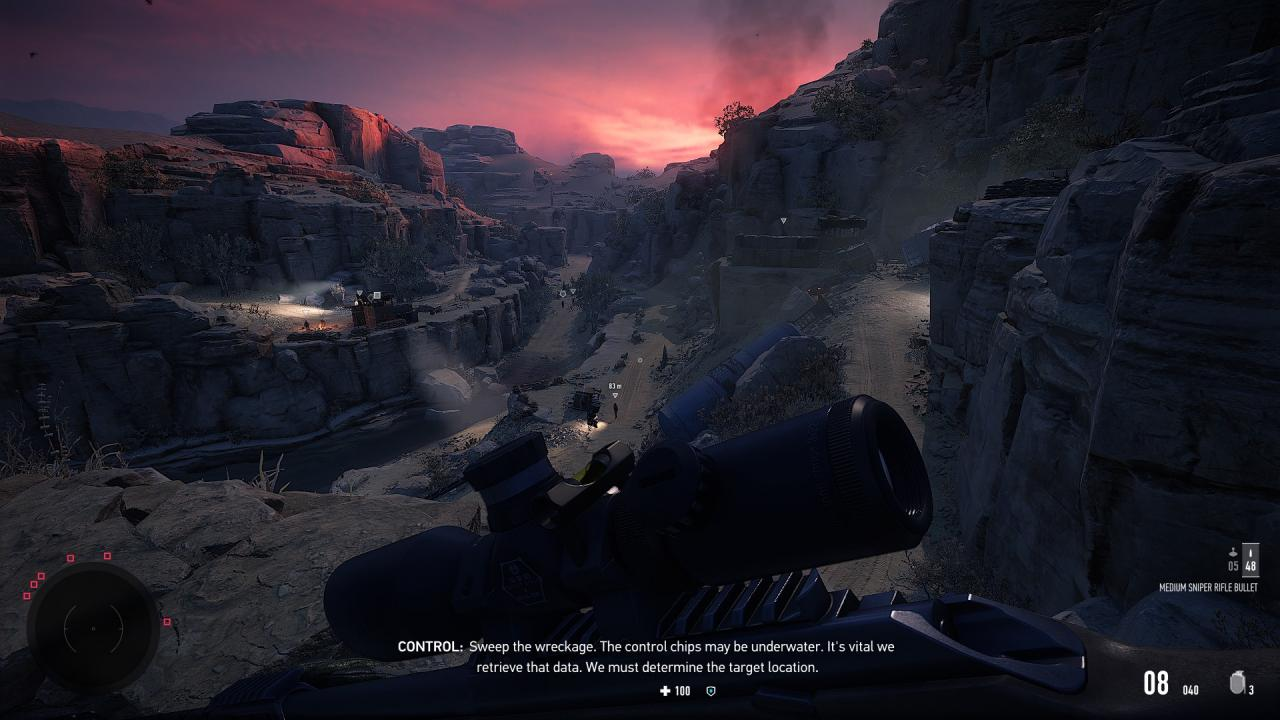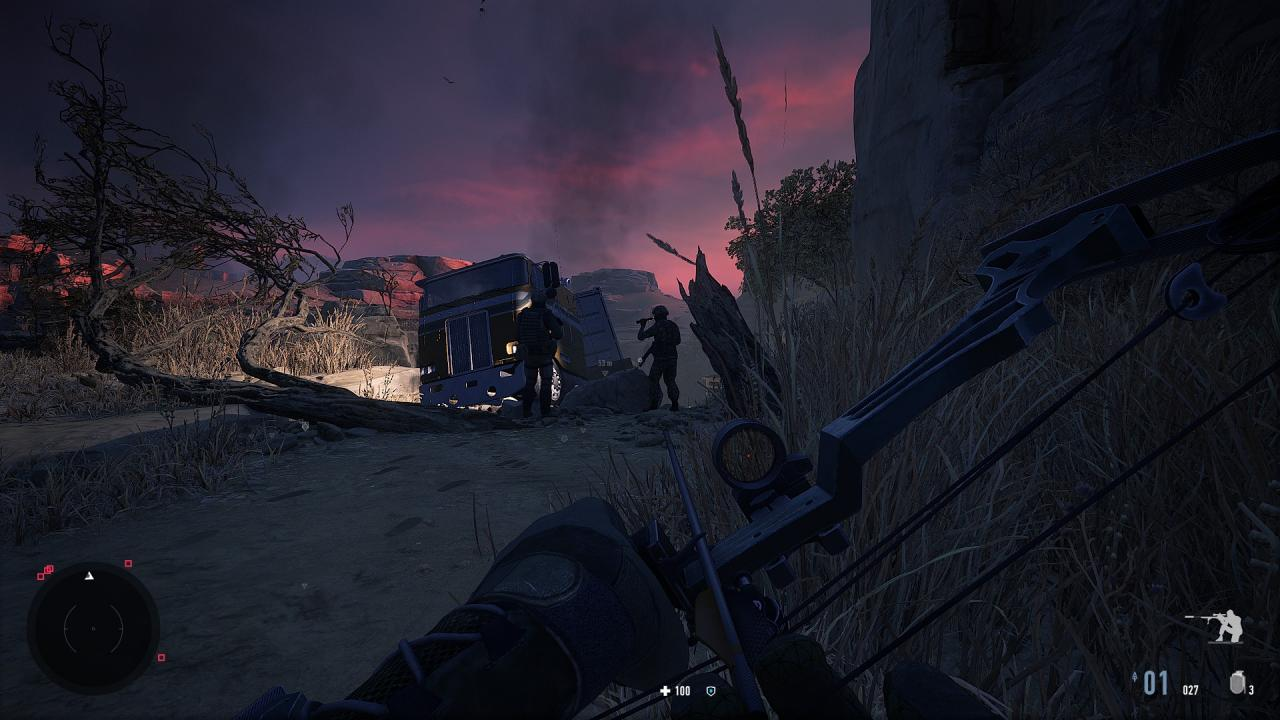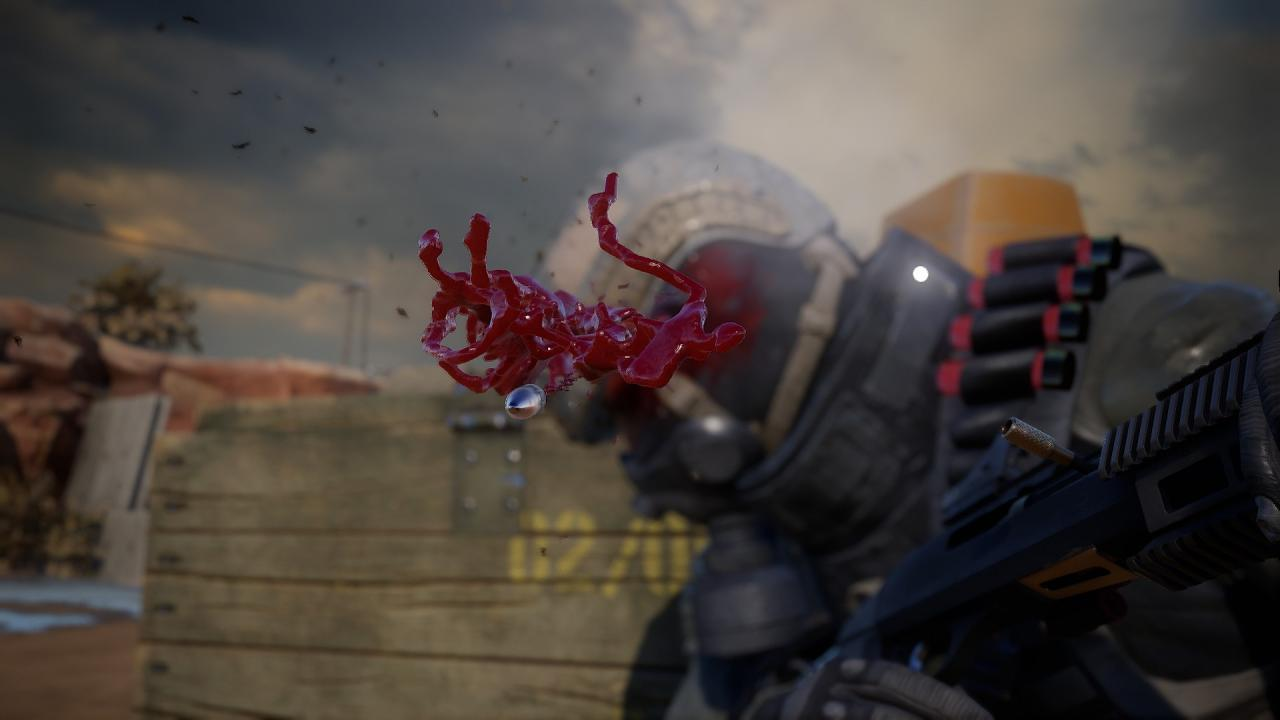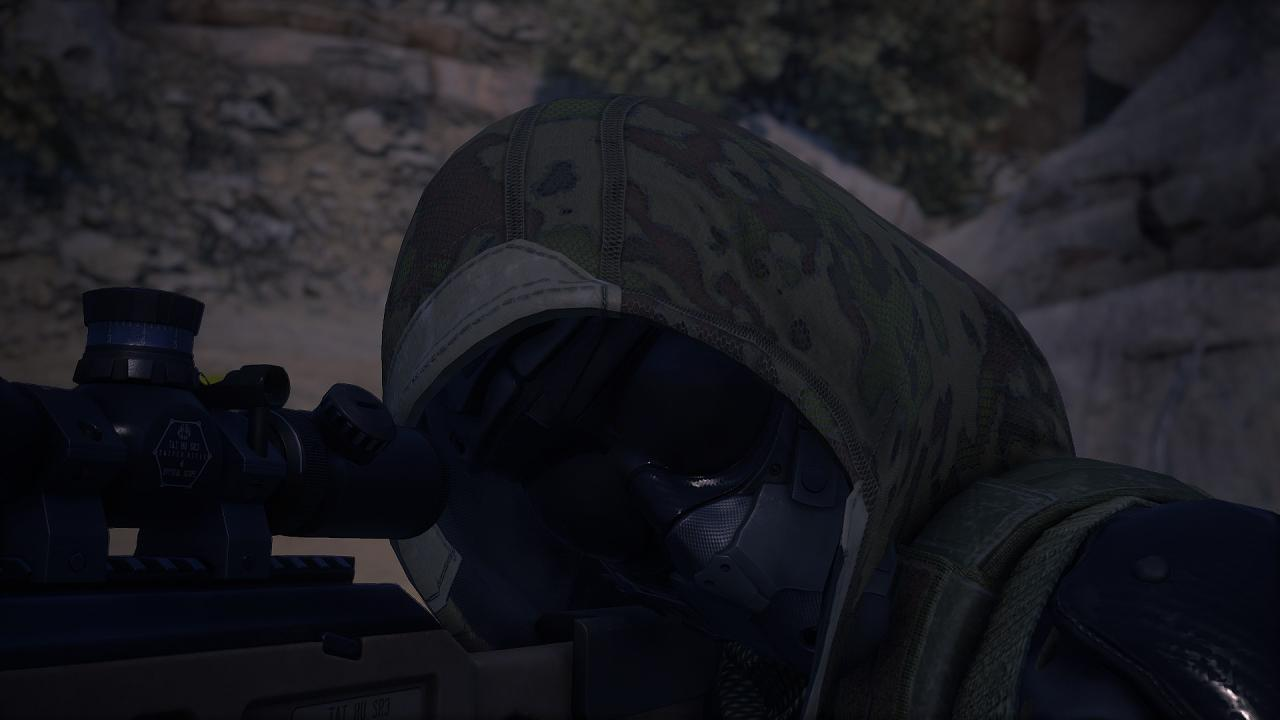# Sniper Ghost Warrior Contracts 2: Graphical Fidelity Settings

Improved Graphical Fidelity Settings for Sniper Ghost Warrior Contracts 2.

This guide will alter settings in an Autoexec.cfg file in order to achieve better graphical fidelity in the game.

I created a settings for SGW 3 and Sniper Ghost Warrior Contracts 1, and am now including the same settings for Sniper Ghost Warrior Contracts 2.

These settings will require appropriate PC hardware in order to achieve the intended results. Therefore, it is recommended that your system requirements meet the Recommended System Requirements for the game.

These settings will push your GPU to the limit, but will result in a better, sharper image.

### Autoexec.cfg Contents

Copy and paste the following into an autoexec.cfg in the game main folder.

Once copy and pasted, save the file, and change its properties to “read only”.

Launch the game, and watch your GPU melt.

```sys_spec = 4
sys_spec_ObjectDetail = 4
sys_spec_Full = 4
sys_spec_GameEffects = 4
sys_spec_MotionBlur = 4
sys_spec_ObjectDetail = 4
sys_spec_Particles = 4
sys_spec_Physics = 4
sys_spec_PostProcessing = 4
sys_spec_Sound = 4
sys_spec_Texture = 4
sys_spec_TextureResolution = 4
sys_spec_VolumetricEffects = 4
sys_spec_Quality = 4
sys_spec_Water = 4
sys_spec_light = 4
sys_spec_RayTracing = 4

;Lights and Global Illumination
e_DynamicLights = 1
e_StatObjTestOBB = 1
e_GI = 1
e_GIAmount = 0.6
e_GIMaxDistance = 100
e_GIIterations = 32
e_LightVolumes = 1
e_GIGlossyReflections = 1
e_GIMaxDistance = 150
e_GIRSMSize = 512
e_GISecondaryOcclusion = 1
e_Gsm_Cache = 1
r_Beams = 1
r_BeamsDistFactor = 0.01
r_BeamsHelpers = 1
r_BeamsMaxSlices = 200

;Clouds
e_Clouds = 1
r_CloudsUpdateAlways = 1
e_SkyType = 1
e_SkyUpdateRate = 100
e_SkyQuality = 1
e_SkyBox = 1
e_Sun = 1
r_SunShafts = 2
e_TimeOfDaySpeed = 0.1

;Color
r_ColorBits = 32
r_DetailDistance = 20
r_DetailNumLayers = 2
r_DepthBits = 32
r_CloakHeatScale = 0.25
r_CloakLightScale = 0.25

;Textures
r_TexAnisotropy = 16
r_TexMaxAnisotropy = 16
r_TexMinAnisotropy = 16
r_TexSkyResolution = 2
r_TexturesStreamingMipBias = -4
r_TexturesStreamPoolSize = 3072
r_DynTexAtlasCloudsMaxSize = 32
r_DynTexAtlasSpritesMaxSize = 32
r_DynTexMaxSize = 80
r_EnvCMResolution = 2
r_EnvTexResolution =
r_TexNoAnisoAlphaTest = 0
r_texturesHighResMips = 2

;Fog
e_Fog = 1
r_FogDepthTest = 0

;Foliage, Vegetation and Terrain
e_ViewDistRatioDetail = 125
e_ViewDistRatio = 125
e_ViewDistRatioVegetation = 125
e_ViewDistMin = 10
e_ViewDistRatioLights = 75
e_TerrainOcclusionCullingVersion = 1
e_PhysFoliage = 2
r_VegetationSpritesTexRes = 64
e_VegetationAlphaBlend = 1
e_LodRatio = 40

;Lights and Global Illumination
e_DynamicLights = 1
e_GIGlossyReflections = 1
r_FlareHqShafts = 1
r_Beams = 1
r_BeamsDistFactor = 0.01
r_BeamsHelpers = 1
r_BeamsMaxSlices = 200
r_BeamsSoftClip = 0
r_Glow = 1
r_FlareHqShafts = 1
r_Flares = 1
r_FlaresChromaShift = 6
r_FlaresTessellationRatio = 1
r_FlaresIrisShaftMaxPolyNum = 0
r_LightPropagationVolumes = 1

;Anti Aliasing
r_MSAA = 1
r_MSAA_quality = 16
r_MSAA_samples = 16
r_PostMSAA = 1
r_PostMSAAMode = 2
r_AntialiasingMode = 3
r_AntialiasingTAAMode = 4
r_AntialiasingTAAPattern = 4
r_AntialiasingTSAAMipBias = -3
r_AntialiasingTSAASubpixelDetection = 1
r_AntialiasingTSAASmoothness = 0.15
r_AntialiasingTAASharpening = 1
r_AntialiasingModeSCull = 1
r_AntialiasingTAAFalloffHiFreq = 0.5
r_AntialiasingTAAFalloffLowFreq = 0.3
r_AntialiasingTAAPattern = 2
r_UseEdgeAA = 2
r_StencilBits = 8
r_AntialiasingSMAAThreshold = 0.1

r_Reflections = 1
q_Quality = 3
q_Renderer = 3
r_TranspDepthFixup = 1

;PostProcessing
r_PostProcessEffectsFilters = 1
r_PostProcessEffects = 1
r_PostProcessFilters = 1
r_PostProcessEffectsGameFx = 1
r_PostProcessGameFx = 1
r_PostProcessHUD3D = 1
r_PostProcessHUD3DCache = 1

;Lighting
r_silhouettePOM = 1
r_UsePOM = 1

;Sun
r_SunShafts = 2
r_Coronas = 1
r_CoronaSizeScale = 1
r_CoronaColorScale = 1
e_Sun = 1

;Rain
r_RainDropsEffect = 1
r_Rain = 2
r_RainMaxViewDist = 100
r_RainMaxViewDist_Deferred = 200
r_RainGBuffer = 1
r_RainOccluderSizeTreshold

r_ChromaticAberration = 1.5
r_Supersampling = 1.99
r_SupersamplingFilter = 1
r_Sharpening = 5
r_ssdo = 2
r_SSReflections = 3
r_Refraction = 1
r_ssdoAmbientAmount = 1
r_ssdoAmountDirect = 1.5
r_ssdoAmountReflection = 1.5

r_DrawNearZRange = 1

;MotionBlur
r_MotionBlurMaxViewDist = 32
r_MotionBlurQuality = 2
r_MotionBlurShutterSpeed = 125
r_PostProcessHUD3DCache = 0

;Depth of Field
r_DepthOfField = 2
r_DepthOfFieldDilation = 0
r_DepthOfFieldBokeh = 1
r_DepthOfFieldBokehQuality = 1
r_DrawNearFoV = 60
r_DrawNearZRange = 0.1
cl_fov = 70

r_overrideRefreshRate = 60
ti_UseApiBackedRaytracing = 1
e_StreamCgfPoolSize = 2048
e_Wind = 1
sys_budget_sysmem = 16384
sys_streaming_CPU = 6
sys_budget_videomem = 6144